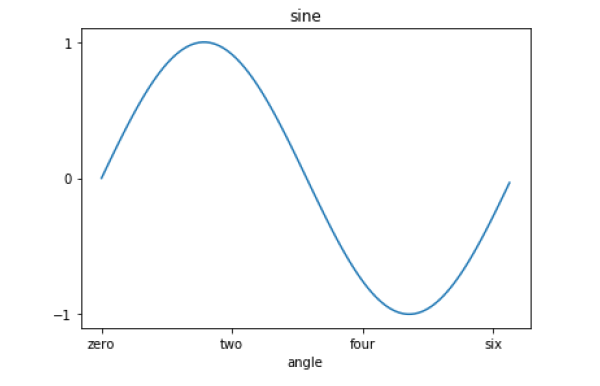# Matplotlib - Setting Ticks and Tick Labels

Advertisements

Ticks are the markers denoting data points on axes. Matplotlib has so far - in all our previous examples - automatically taken over the task of spacing points on the axis.Matplotlib's default tick locators and formatters are designed to be generally sufficient in many common situations. Position and labels of ticks can be explicitly mentioned to suit specific requirements.

The xticks() and yticks() function takes a list object as argument. The elements in the list denote the positions on corresponding action where ticks will be displayed.

```ax.set_xticks([2,4,6,8,10])
```

This method will mark the data points at the given positions with ticks.

Similarly, labels corresponding to tick marks can be set by set_xlabels() and set_ylabels() functions respectively.

```ax.set_xlabels([‘two’, ‘four’,’six’, ‘eight’, ‘ten’])
```

This will display the text labels below the markers on the x axis.

Following example demonstrates the use of ticks and labels.

```import matplotlib.pyplot as plt
import numpy as np
import math
x = np.arange(0, math.pi*2, 0.05)
fig = plt.figure()
ax = fig.add_axes([0.1, 0.1, 0.8, 0.8]) # main axes
y = np.sin(x)
ax.plot(x, y)
ax.set_xlabel(‘angle’)
ax.set_title('sine')
ax.set_xticks([0,2,4,6])
ax.set_xticklabels(['zero','two','four','six'])
ax.set_yticks([-1,0,1])
plt.show()
```Advertisements